# Shapes#

## Introduction#

CrSFML provides a set of classes that represent simple shape entities. Each type of shape is a separate class, but they all derive from the same base class so that they have access to the same subset of common features. Each class then adds its own specifics: a radius property for the circle class, a size for the rectangle class, points for the polygon class, etc.

## Common shape properties#

### Transformation (position, rotation, scale)#

These properties are common to all the CrSFML graphical classes, so they are explained in a separate tutorial: Transforming entities.

### Color#

One of the basic properties of a shape is its color. You can change with the `fill_color=` method.

``````shape = SF::CircleShape.new(50)

# set the shape color to green
shape.fill_color = SF.color(100, 250, 50)
``````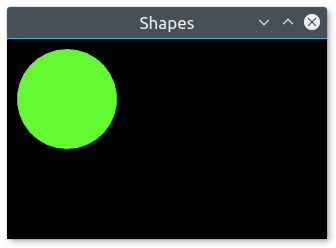### Outline#

Shapes can have an outline. You can set the thickness and color of the outline with the `outline_thickness=` and `outline_color=` methods.

``````shape = SF::CircleShape.new(50)
shape.fill_color = SF.color(150, 50, 250)

# set a 10-pixel wide orange outline
shape.outline_thickness = 10
shape.outline_color = SF.color(250, 150, 100)
``````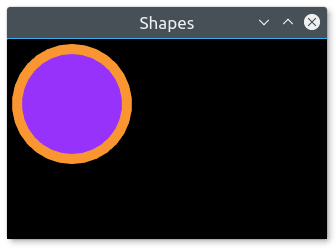By default, the outline is extruded outwards from the shape (e.g. if you have a circle with a radius of 10 and an outline thickness of 5, the total radius of the circle will be 15). You can make it extrude towards the center of the shape instead, by setting a negative thickness.

To disable the outline, set its thickness to 0. If you only want the outline, you can set the fill color to `SF::Color::Transparent`.

### Texture#

Shapes can also be textured, just like sprites. To specify a part of the texture to be mapped to the shape, you must use the `texture_rect=` method. It takes the texture rectangle to map to the bounding rectangle of the shape. This method doesn't offer maximum flexibility, but it is much easier to use than individually setting the texture coordinates of each point of the shape.

``````shape = SF::CircleShape.new(50)

# map a 100x100 textured rectangle to the shape
shape.set_texture(texture, reset_rect: false) # texture is a SF::Texture
shape.texture_rect = SF.int_rect(10, 10, 100, 100)
``````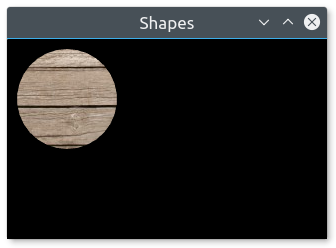Note that the outline is not textured. It is important to know that the texture is modulated (multiplied) with the shape's fill color. If its fill color is `SF::Color::White`, the texture will appear unmodified. To disable texturing, call `set_texture(nil, false)`.

## Drawing a shape#

Drawing a shape is as simple as drawing any other SFML entity:

``````window.draw(shape)
``````

## Built-in shape types#

### Rectangles#

To draw rectangles, you can use the SF::RectangleShape class. It has a single attribute: The size of the rectangle.

``````# define a 120x50 rectangle
rectangle = SF::RectangleShape.new(SF.vector2(120, 50))

# change the size to 100x100
rectangle.size = SF.vector2(100, 100)
``````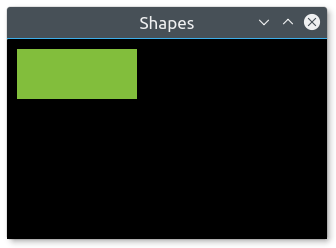### Circles#

Relevant example: snakes

Circles are represented by the SF::CircleShape class. It has two attributes: The radius and the number of sides. The number of sides is an optional attribute, it allows you to adjust the "quality" of the circle: Circles have to be approximated by polygons with many sides (the graphics card is unable to draw a perfect circle directly), and this attribute defines how many sides your circle approximation will have. If you draw small circles, you'll probably only need a few sides. If you draw big circles, or zoom on regular circles, you'll most likely need more sides.

``````# define a circle with radius = 200
circle = SF::CircleShape.new(200)

# change the radius to 40

# change the number of sides (points) to 100
circle.point_count = 100
``````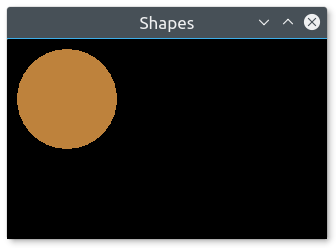### Regular polygons#

There's no dedicated class for regular polygons, in fact you can represent a regular polygon with any number of sides using the SF::CircleShape class: Since circles are approximated by polygons with many sides, you just have to play with the number of sides to get the desired polygons. A SF::CircleShape with 3 points is a triangle, with 4 points it's a square, etc.

``````# define a triangle
triangle = SF::CircleShape.new(80, 3)

# define a square
square = SF::CircleShape.new(80, 4)

# define an octagon
octagon = SF::CircleShape.new(80, 8)
``````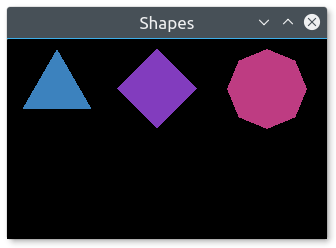### Convex shapes#

Relevant example: rounded_rectangle

The SF::ConvexShape class is the ultimate shape class: It allows you to define any convex shape. SFML is unable to draw concave shapes. If you need to draw a concave shape, you'll have to split it into multiple convex polygons.

To construct a convex shape, you must first set the number of points it should have and then define the points.

``````# create an empty shape
convex = SF::ConvexShape.new

# resize it to 5 points
convex.point_count = 5

# define the points
convex = SF.vector2(0, 0)
convex = SF.vector2(150, 10)
convex = SF.vector2(120, 90)
convex = SF.vector2(30, 100)
convex = SF.vector2(0, 50)
``````

The order in which you define the points is very important. They must all be defined either in clockwise or counter-clockwise order. If you define them in an inconsistent order, the shape will be constructed incorrectly.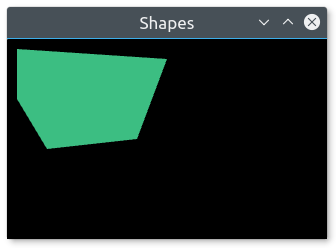Although the name of SF::ConvexShape implies that it should only be used to represent convex shapes, its requirements are a little more relaxed. In fact, the only requirement that your shape must meet is that if you went ahead and drew lines from its center of gravity to all of its points, these lines must be drawn in the same order. You are not allowed to "jump behind a previous line". Internally, convex shapes are automatically constructed using triangle fans, so if your shape is representable by a triangle fan, you can use SF::ConvexShape. With this relaxed definition, you can draw stars using SF::ConvexShape for example.

### Lines#

There's no shape class for lines. The reason is simple: If your line has a thickness, it is a rectangle. If it doesn't, it can be drawn with a line primitive.

Line with thickness:

``````line = SF::RectangleShape.new(SF.vector2(150, 5))
line.rotate(45)
``````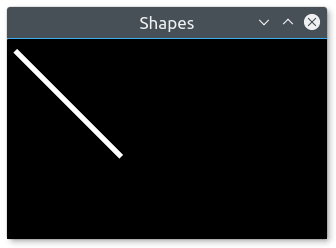Line without thickness:

``````line = [
SF::Vertex.new(SF.vector2(10, 10)),
SF::Vertex.new(SF.vector2(150, 150))
]

window.draw(line, SF::Lines)
``````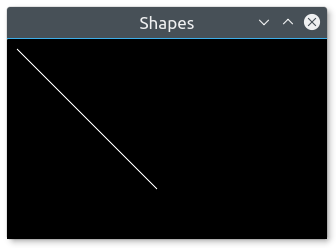## Custom shape types#

Relevant example: shapes

You can extend the set of shape classes with your own shape types. To do so, you must derive from SF::Shape and override two methods:

• `point_count`: return the number of points in the shape
• `get_point`: return a point of the shape

You must also call the `update()` method whenever any point in your shape changes, so that the base class is informed and can update its internal geometry.

Here is a complete example of a custom shape class: `EllipseShape`.

``````class EllipseShape < SF::Shape
super()
update()
end

end
update
end

def point_count
40  # fixed, but could be an attribute of the class if needed
end
def get_point(index)
angle = index * 2*Math::PI / point_count

# origin is {0, 0}, center is @radius
end
end
``````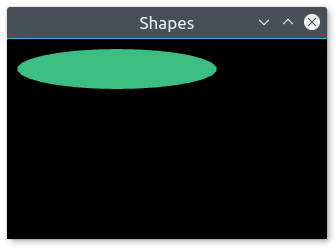## Antialiased shapes#

Relevant example: snakes

There's no option to anti-alias a single shape. To get anti-aliased shapes (i.e. shapes with smoothed edges), you have to enable anti-aliasing globally when you create the window, with the corresponding attribute of the structure.

``````window = SF::RenderWindow.new(
SF::VideoMode.new(800, 600),
"SFML shapes",
settings: SF::ContextSettings.new(depth: 24, antialiasing: 8)
)
``````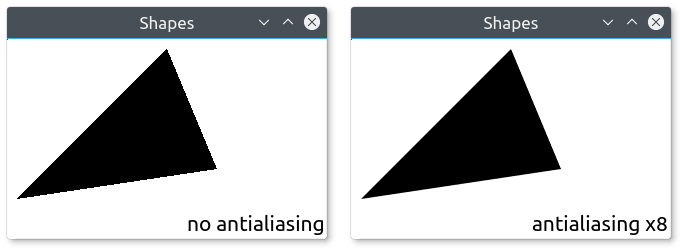Remember that anti-aliasing availability depends on the graphics card: It might not support it, or have it forced to disabled in the driver settings. Sometimes antialiasing does not work if `depth_bits` is not specified (use 24 if unsure).